When using polygon assets we can only draw one object at a time (ignoring stuff like batching and instancing), but when working with signed distance fields we aren’t bound by the same limitations, if two positions have the same coordinate, the signed distance functions will return the same value and you can get multiple shapes with one calculation. To learn how to transform the space we use to generate signed distance fields I recommend you understand how to create shapes with signed distance functions and combine sdf shapes.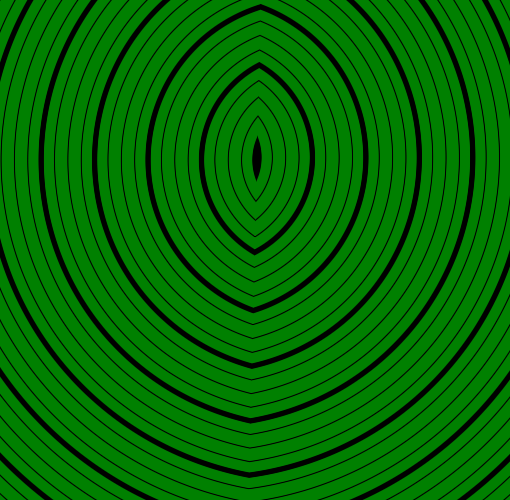## Setup

For this tutorial I’ll modify a union between a square and a circle, but you can use it on any shape you want. It’s similar to the setup for the previous tutorial.

Important here is that the part we will modify is before we use the position to generate shapes.

``````Shader "Tutorial/036_SDF_Space_Manpulation/Type"{
Properties{
_InsideColor("Inside Color", Color) = (.5, 0, 0, 1)
_OutsideColor("Outside Color", Color) = (0, .5, 0, 1)

_LineDistance("Mayor Line Distance", Range(0, 2)) = 1
_LineThickness("Mayor Line Thickness", Range(0, 0.1)) = 0.05

[IntRange]_SubLines("Lines between major lines", Range(1, 10)) = 4
_SubLineThickness("Thickness of inbetween lines", Range(0, 0.05)) = 0.01
}

//the material is completely non-transparent and is rendered at the same time as the other opaque geometry
Tags{ "RenderType"="Opaque" "Queue"="Geometry"}

Pass{
CGPROGRAM

#include "UnityCG.cginc"
#include "2D_SDF.cginc"

#pragma vertex vert
#pragma fragment frag

struct appdata{
float4 vertex : POSITION;
};

struct v2f{
float4 position : SV_POSITION;
float4 worldPos : TEXCOORD0;
};

v2f vert(appdata v){
v2f o;
//calculate the position in clip space to render the object
o.position = UnityObjectToClipPos(v.vertex);
//calculate world position of vertex
o.worldPos = mul(unity_ObjectToWorld, v.vertex);
return o;
}

float scene(float2 position) {

// manipulate position with cool methods here!

float2 squarePosition = position;
squarePosition = translate(squarePosition, float2(2, 2));
squarePosition = rotate(squarePosition, .125);
float squareShape = rectangle(squarePosition, float2(1, 1));

float2 circlePosition = position;
circlePosition = translate(circlePosition, float2(1, 1.5));
float circleShape = circle(circlePosition, 1);

float combination = merge(circleShape, squareShape);

return combination;
}

float4 _InsideColor;
float4 _OutsideColor;

float _LineDistance;
float _LineThickness;

float _SubLines;
float _SubLineThickness;

fixed4 frag(v2f i) : SV_TARGET{
float dist = scene(i.worldPos.xz);
fixed4 col = lerp(_InsideColor, _OutsideColor, step(0, dist));

float distanceChange = fwidth(dist) * 0.5;
float majorLineDistance = abs(frac(dist / _LineDistance + 0.5) - 0.5) * _LineDistance;
float majorLines = smoothstep(_LineThickness - distanceChange, _LineThickness + distanceChange, majorLineDistance);

float distanceBetweenSubLines = _LineDistance / _SubLines;
float subLineDistance = abs(frac(dist / distanceBetweenSubLines + 0.5) - 0.5) * distanceBetweenSubLines;
float subLines = smoothstep(_SubLineThickness - distanceChange, _SubLineThickness + distanceChange, subLineDistance);

return col * majorLines * subLines;
}

ENDCG
}
}
FallBack "Standard"
}
``````

And the 2D_SDF.cginc function in the same folder as the shader we will expand looks like this at the start:

``````#ifndef SDF_2D
#define SDF_2D

//transforms

float2 rotate(float2 samplePosition, float rotation){
const float PI = 3.14159;
float angle = rotation * PI * 2 * -1;
float sine, cosine;
sincos(angle, sine, cosine);
return float2(cosine * samplePosition.x + sine * samplePosition.y, cosine * samplePosition.y - sine * samplePosition.x);
}

float2 translate(float2 samplePosition, float2 offset){
//move samplepoint in the opposite direction that we want to move shapes in
return samplePosition - offset;
}

float2 scale(float2 samplePosition, float scale){
return samplePosition / scale;
}

//combinations

///basic
float merge(float shape1, float shape2){
return min(shape1, shape2);
}

float intersect(float shape1, float shape2){
return max(shape1, shape2);
}

float subtract(float base, float subtraction){
return intersect(base, -subtraction);
}

float interpolate(float shape1, float shape2, float amount){
return lerp(shape1, shape2, amount);
}

/// round
float round_merge(float shape1, float shape2, float radius){
intersectionSpace = min(intersectionSpace, 0);
float insideDistance = -length(intersectionSpace);
float simpleUnion = merge(shape1, shape2);
return  insideDistance + outsideDistance;
}

float round_intersect(float shape1, float shape2, float radius){
intersectionSpace = max(intersectionSpace, 0);
float outsideDistance = length(intersectionSpace);
float simpleIntersection = intersect(shape1, shape2);
return outsideDistance + insideDistance;
}

float round_subtract(float base, float subtraction, float radius){
}

///champfer
float champfer_merge(float shape1, float shape2, float champferSize){
const float SQRT_05 = 0.70710678118;
float simpleMerge = merge(shape1, shape2);
float champfer = (shape1 + shape2) * SQRT_05;
champfer = champfer - champferSize;
return merge(simpleMerge, champfer);
}

float champfer_intersect(float shape1, float shape2, float champferSize){
const float SQRT_05 = 0.70710678118;
float simpleIntersect = intersect(shape1, shape2);
float champfer = (shape1 + shape2) * SQRT_05;
champfer = champfer + champferSize;
return intersect(simpleIntersect, champfer);
}

float champfer_subtract(float base, float subtraction, float champferSize){
return champfer_intersect(base, -subtraction, champferSize);
}

/// round border intersection
float round_border(float shape1, float shape2, float radius){
float2 position = float2(shape1, shape2);
float distanceFromBorderIntersection = length(position);
}

float groove_border(float base, float groove, float width, float depth){
float circleBorder = abs(groove) - width;
float grooveShape = subtract(circleBorder, base + depth);
return subtract(base, grooveShape);
}

//shapes

//get distance from center and grow it according to radius
}

float rectangle(float2 samplePosition, float2 halfSize){
float2 componentWiseEdgeDistance = abs(samplePosition) - halfSize;
float outsideDistance = length(max(componentWiseEdgeDistance, 0));
float insideDistance = min(max(componentWiseEdgeDistance.x, componentWiseEdgeDistance.y), 0);
return outsideDistance + insideDistance;
}

#endif
``````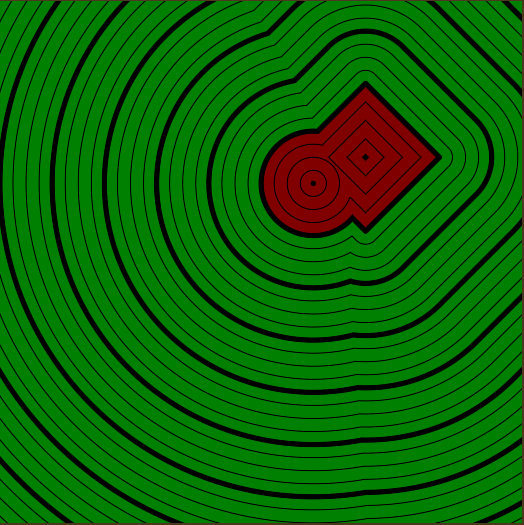## Space Repetition

### Mirror

One of the simplest things we can do is to mirror the world around a axis. To mirror it around the y axis we take the absolute value of the x component of our position. This way the coordinates on the right and the left of the axis are the same. `(-1, 1)` becomes `(1, 1)` and by that it’s inside a circle that uses `(1, 1)` as it’s origin with a radius greater than 0.

Because most of the time the code using this function would look like `position = mirror(position);` anyways, we can use a small shortcut here. We simply declare the position argument as inout. This way when we write to the argument it’ll also change the variable where we pass it into the function. The return type can then be void because we’re not using the return value anyways.

``````//in 2D_SDF.cginc

void mirror(inout float2 position){
position.x = abs(position.x);
}
``````
``````//in shader function

mirror(position);
``````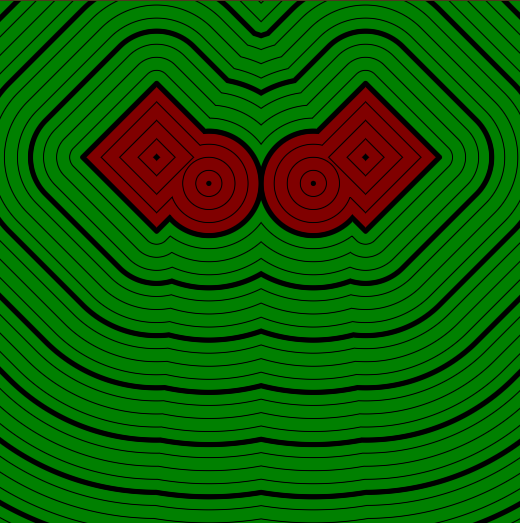This is pretty nice already, but it only gives us a single axis to mirror around. We can expand that by rotating the space just like we did for rotating shapes. We first have to rotate the space, then mirror it and then rotate it back. This way we can mirror around any angle. The same is possible by translating the space and doing the inverse translation after mirroring. (If you’re doing both, remember to first translate, then rotate before mirroring and rotating first afterwards)

``````//in shader function

float rotation = _Time.y * 0.25;
position = rotate(position, rotation);
mirror(position);
position = rotate(position, -rotation);
``````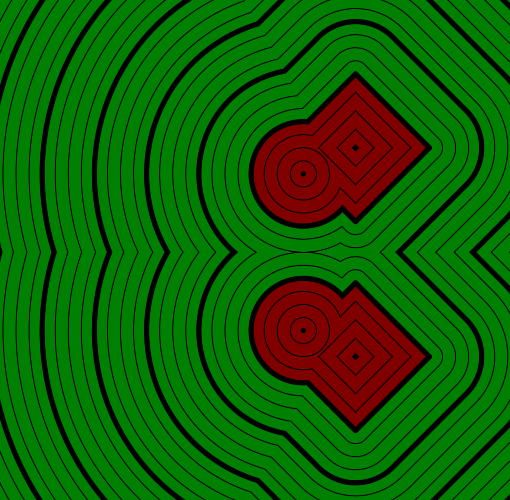## Cells

If you know how generating noise works, you know that for procedural generation we often repeat the position and have small cells which are essentially the same except for a few paramters. For distance fields we can do the same.

Because the `fmod` function (as well as using % for the modulo) gives us the remainder instead of the definition of a modulo we want we’ll have to use some trickery. We first take the modulo with the fmod function. For positive numbers that’s what we want, for negative numbers though, it’s the result we want minus the period. The fix for this is to add the period and take the modulo again. Adding the period will give us the result we want for negative input values and a value that’s one period too high for positive input values. The second modulo will do nothing to the values for negative input values, because they’re already between 0 and the period, for positive input values, it’ll basically subtract one period.

``````//in 2D_SDF.cginc

void cells(inout float2 position, float2 period){
position = fmod(position, period);
//negative positions lead to negative modulo
position += period;
//negative positions now have correct cell coordinates, positive input positions too high
position = fmod(position, period);
//second mod doesn't change values between 0 and period, but brings down values that are above period.
}
``````
``````//in shader function

cells(position, float2(3, 3));
``````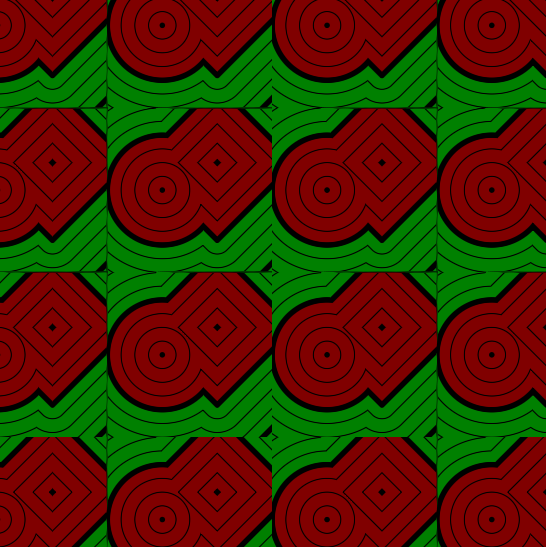A problem with cells is that we loose the continuity we like in distance fields. It’s not that bad when the shapes are only in the middle of the cells, but in examples like the one I have here, it can lead to massive artefacts we want to avoid if we use the distance field for many things we might want to use distance fields for.

One solution that doesn’t work in every case, but which is great where it works is to mirror every second cell. For this we need the cell index of our pixel, but we still have no return value in our function, so we can easily use that to return the cell index.

To calculate the cell index we divide the position by the period. this way 0-1 is the first cell, 1-2 the second etc… and we can easily quantise this. To get the cell index we then simply floor the value and return the result. It’s important that we calculate the cell index before we do the modulo to repeat the cells, otherwise we’d get a index of 0 everywhere because the position doesn’t go above the period.

``````//in 2D_SDF.cginc

float2 cells(inout float2 position, float2 period){
position = fmod(position, period);
//negative positions lead to negative modulo
position += period;
//negative positions now have correct cell coordinates, positive input positions too high
position = fmod(position, period);
//second mod doesn't change values between 0 and period, but brings down values that are above period.

float2 cellIndex = position / period;
cellIndex = floor(cellIndex);
return cellIndex;
}
``````

With this information we can now flip the cells. To get whether we should or should not flip, we take the modulo of 2 with the cell index. The result of this operations changes between 0 and 1 or -1 every second cell. To make the changing more consistent, we take the sbolute value and have a value that switches between 0 and 1.

To use this value to flip between the normal position and the flipped one we need a function that does nothing for a value of 0 and subtracts the position from the period where flip is 1. So we do a linear interpolation from the normal position to the flipped one via the flip variable. Because the flip variable is a 2d vector the components are individually flipped.

``````//in shader function

float2 period = 3;
float2 cell = cells(position, period);
float2 flip = abs(fmod(cell, 2));
position = lerp(position, period - position, flip);
``````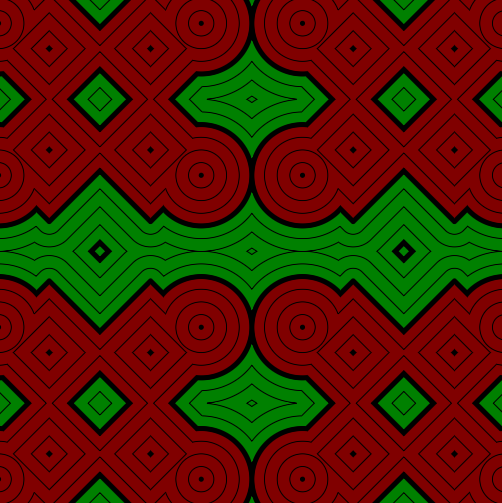Another cool thing is to repeat the space in a radial pattern.

To get that effect, we first calculate the radial position. For that we encode the angle around the center in the x axis and the distance from the center in the y axis.

``````float2 radialPosition = float2(atan2(position.x, position.y), length(position));
``````

Then we repeat the angle. Because passing in the amount of repetitions is way easier than the angle of each slice we first calculate the size of each slice. A whole circle is 2 times pi, so to get the part we want we divide 2 times pi by the cell amount.

``````const float PI = 3.14159;
float cellSize = PI * 2 / cells;
``````

With this infomation we can now repeat the x component of the radial position every cellSize units. We do the repetition via the modulo, just like before we get problems with negative numbers here which we have to mitigate by using two modulo functions.

``````radialPosition.x = fmod(fmod(radialPosition.x, cellSize) + cellSize, cellSize);
``````

Then we have to transfer the new position back into normal xy coordinates. We use the sincos function with the x component of the radial position as the angle here to write the sine into the x coordinate of the position and the cosine into the y coordinate. With this step we get the normalised position. To get the correct distance from the center we then have to multiply it by the y component of the radial position, which signifies the length.

``````//in 2D_SDF.cginc

void radial_cells(inout float2 position, float cells){
const float PI = 3.14159;

float cellSize = PI * 2 / cells;
float2 radialPosition = float2(atan2(position.x, position.y), length(position));

}
``````
``````//in shader function

float2 period = 6;
``````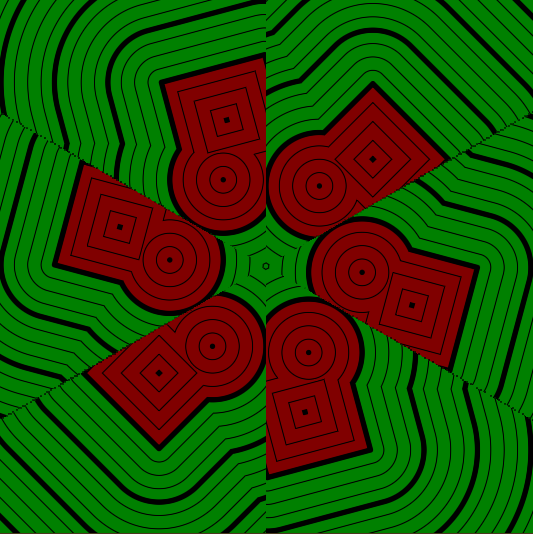Then we can also add a cell index and mirroring just like we did for the regular cells.

We have to calculate the cell index after calculating the radial position, but before taking it’s modulo. We get it by dividing the x component of the radial position and flooring the result. In this case the index can also be negative, that’s a problem if we have a uneven amount of cells. For example with 3 cells, we’d get 1 cell with index 0, 1 cell with a index of -1 and 2 half cells with each 1 and -2. To sidestep this problem, we add the amount of cells to the floored variable and then take a modulo with the cellsize.

``````//in 2D_SDF.cginc

float cellIndex = fmod(floor(radialPosition.x / cellSize) + cells, cells);

//at the end of the function:
return cellIndex;
``````

To mirror this, we’d like to have the coordinates as radial coordinates, so to avoid calculating the radial coordinates again outside of the function we’re going to give the option via a bool argument. Usually we really don’t like having branching (if statements) in our shaders, but in this case all pixels on the screen will take the same path, so it’s fine.

The mirroring has to happen after the radial coordinate was looped, but before it’s transformed back into a regular position. We get whether the current cell should be flipped or not by taking the modulo of the cell index and 2. This usually should give us zeroes and ones, but in my case I experienced some twos, which is weird, but we can work with. To fix the twos, we simply subtract one 1 from our flip variable and then take the absolute value, this way zeroes and twos become ones and the ones become zero, just like we like it, just the other way around.

Because the zeroes and ones are the wrong way around, we do a linear interpolation from the flipped version to the unflipped one, not the other way around that we did previously. To flip the coordinate we just subtract the position from the cell size.

``````//in 2D_SDF.cginc

float radial_cells(inout float2 position, float cells, bool mirrorEverySecondCell = false){
const float PI = 3.14159;

float cellSize = PI * 2 / cells;
float2 radialPosition = float2(atan2(position.x, position.y), length(position));

float cellIndex = fmod(floor(radialPosition.x / cellSize) + cells, cells);

if(mirrorEverySecondCell){
float flip = fmod(cellIndex, 2);
flip = abs(flip-1);
}

return cellIndex;
}
``````
``````//in shader function

float2 period = 6;
``````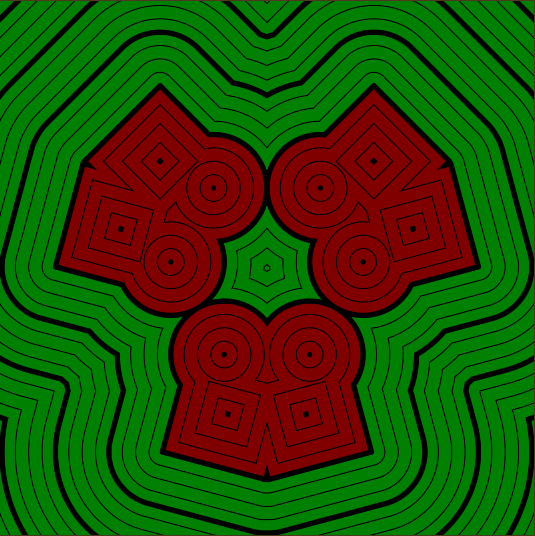## Wobbly space

But we don’t have to repeat the space to change it. In the tutorial about basics we rotate, transform and scale it for example. Another thing we can do is to move each axis based on the other one with a sine wave. This does make the distances of the signed distance funciton less precise, but as long as we don’t make it wobble too much it should be fine.

We first calculate the amount we change the position by flipping the x and y components and then multiplying them by the frequency of the wobble. Then we take the sine of that value and multiply it by the amount of wobble we want to add. After that we simply add that wobble factor to the position and apply the result to the position again.

``````//in 2D_SDF.cginc

void wobble(inout float2 position, float2 frequency, float2 amount){
float2 wobble = sin(position.yx * frequency) * amount;
position = position + wobble;
}
``````
``````//in shader function

wobble(position, 5, .05);
``````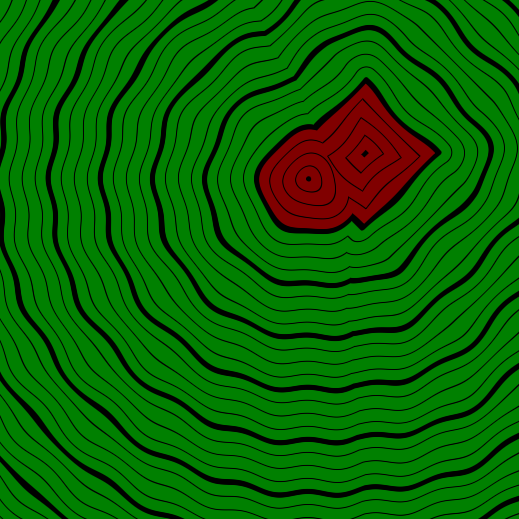We can also animate that wobble by changing the position, applying the wobble at the offset position and moving the space back. To avoid the float numbers from becomming too big and creating ugly artefacts, I do a modulo at pi times 2 divided by the frequency of the wobble, this lines up with the wobble (a sine wave repeats every pi times 2 units) so you won’t see the jump and prevents the offset from becoming too big.

``````//in shader function
const float PI = 3.14159;

float frequency = 5;
float offset = _Time.y;
offset = fmod(offset, PI * 2 / frequency);
position = translate(position, offset);
wobble(position, 5, .05);
position = translate(position, -offset);
``````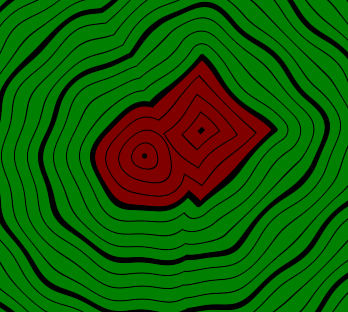## Sources

### 2D SDF Library

``````#ifndef SDF_2D
#define SDF_2D

//transforms

float2 rotate(float2 samplePosition, float rotation){
const float PI = 3.14159;
float angle = rotation * PI * 2 * -1;
float sine, cosine;
sincos(angle, sine, cosine);
return float2(cosine * samplePosition.x + sine * samplePosition.y, cosine * samplePosition.y - sine * samplePosition.x);
}

float2 translate(float2 samplePosition, float2 offset){
//move samplepoint in the opposite direction that we want to move shapes in
return samplePosition - offset;
}

float2 scale(float2 samplePosition, float scale){
return samplePosition / scale;
}

//combinations

///basic
float merge(float shape1, float shape2){
return min(shape1, shape2);
}

float intersect(float shape1, float shape2){
return max(shape1, shape2);
}

float subtract(float base, float subtraction){
return intersect(base, -subtraction);
}

float interpolate(float shape1, float shape2, float amount){
return lerp(shape1, shape2, amount);
}

/// round
float round_merge(float shape1, float shape2, float radius){
intersectionSpace = min(intersectionSpace, 0);
float insideDistance = -length(intersectionSpace);
float simpleUnion = merge(shape1, shape2);
return  insideDistance + outsideDistance;
}

float round_intersect(float shape1, float shape2, float radius){
intersectionSpace = max(intersectionSpace, 0);
float outsideDistance = length(intersectionSpace);
float simpleIntersection = intersect(shape1, shape2);
return outsideDistance + insideDistance;
}

float round_subtract(float base, float subtraction, float radius){
}

///champfer
float champfer_merge(float shape1, float shape2, float champferSize){
const float SQRT_05 = 0.70710678118;
float simpleMerge = merge(shape1, shape2);
float champfer = (shape1 + shape2) * SQRT_05;
champfer = champfer - champferSize;
return merge(simpleMerge, champfer);
}

float champfer_intersect(float shape1, float shape2, float champferSize){
const float SQRT_05 = 0.70710678118;
float simpleIntersect = intersect(shape1, shape2);
float champfer = (shape1 + shape2) * SQRT_05;
champfer = champfer + champferSize;
return intersect(simpleIntersect, champfer);
}

float champfer_subtract(float base, float subtraction, float champferSize){
return champfer_intersect(base, -subtraction, champferSize);
}

/// round border intersection
float round_border(float shape1, float shape2, float radius){
float2 position = float2(shape1, shape2);
float distanceFromBorderIntersection = length(position);
}

float groove_border(float base, float groove, float width, float depth){
float circleBorder = abs(groove) - width;
float grooveShape = subtract(circleBorder, base + depth);
return subtract(base, grooveShape);
}

// space repetition

void mirror(inout float2 position){
position.x = abs(position.x);
}

float2 cells(inout float2 position, float2 period){
//find cell index
float2 cellIndex = position / period;
cellIndex = floor(cellIndex);

//negative positions lead to negative modulo
position = fmod(position, period);
//negative positions now have correct cell coordinates, positive input positions too high
position += period;
//second mod doesn't change values between 0 and period, but brings down values that are above period.
position = fmod(position, period);

return cellIndex;
}

float radial_cells(inout float2 position, float cells, bool mirrorEverySecondCell = false){
const float PI = 3.14159;

float cellSize = PI * 2 / cells;
float2 radialPosition = float2(atan2(position.x, position.y), length(position));

float cellIndex = fmod(floor(radialPosition.x / cellSize) + cells, cells);

if(mirrorEverySecondCell){
float flip = fmod(cellIndex, 2);
flip = abs(flip-1);
}

return cellIndex;
}

void wobble(inout float2 position, float2 frequency, float2 amount){
float2 wobble = sin(position.yx * frequency) * amount;
position = position + wobble;
}

//shapes

//get distance from center and grow it according to radius
}

float rectangle(float2 samplePosition, float2 halfSize){
float2 componentWiseEdgeDistance = abs(samplePosition) - halfSize;
float outsideDistance = length(max(componentWiseEdgeDistance, 0));
float insideDistance = min(max(componentWiseEdgeDistance.x, componentWiseEdgeDistance.y), 0);
return outsideDistance + insideDistance;
}

#endif
``````

``````Shader "Tutorial/036_SDF_Space_Manpulation/Mirror"{
Properties{
_InsideColor("Inside Color", Color) = (.5, 0, 0, 1)
_OutsideColor("Outside Color", Color) = (0, .5, 0, 1)

_LineDistance("Mayor Line Distance", Range(0, 2)) = 1
_LineThickness("Mayor Line Thickness", Range(0, 0.1)) = 0.05

[IntRange]_SubLines("Lines between major lines", Range(1, 10)) = 4
_SubLineThickness("Thickness of inbetween lines", Range(0, 0.05)) = 0.01
}

//the material is completely non-transparent and is rendered at the same time as the other opaque geometry
Tags{ "RenderType"="Opaque" "Queue"="Geometry"}

Pass{
CGPROGRAM

#include "UnityCG.cginc"
#include "2D_SDF.cginc"

#pragma vertex vert
#pragma fragment frag

struct appdata{
float4 vertex : POSITION;
};

struct v2f{
float4 position : SV_POSITION;
float4 worldPos : TEXCOORD0;
};

v2f vert(appdata v){
v2f o;
//calculate the position in clip space to render the object
o.position = UnityObjectToClipPos(v.vertex);
//calculate world position of vertex
o.worldPos = mul(unity_ObjectToWorld, v.vertex);
return o;
}

float scene(float2 position) {

// modify position here!

float2 squarePosition = position;
squarePosition = translate(squarePosition, float2(2, 2));
squarePosition = rotate(squarePosition, .125);
float squareShape = rectangle(squarePosition, float2(1, 1));

float2 circlePosition = position;
circlePosition = translate(circlePosition, float2(1, 1.5));
float circleShape = circle(circlePosition, 1);

float combination = merge(circleShape, squareShape);

return combination;
}

float4 _InsideColor;
float4 _OutsideColor;

float _LineDistance;
float _LineThickness;

float _SubLines;
float _SubLineThickness;

fixed4 frag(v2f i) : SV_TARGET{
float dist = scene(i.worldPos.xz);
fixed4 col = lerp(_InsideColor, _OutsideColor, step(0, dist));

float distanceChange = fwidth(dist) * 0.5;
float majorLineDistance = abs(frac(dist / _LineDistance + 0.5) - 0.5) * _LineDistance;
float majorLines = smoothstep(_LineThickness - distanceChange, _LineThickness + distanceChange, majorLineDistance);

float distanceBetweenSubLines = _LineDistance / _SubLines;
float subLineDistance = abs(frac(dist / distanceBetweenSubLines + 0.5) - 0.5) * distanceBetweenSubLines;
float subLines = smoothstep(_SubLineThickness - distanceChange, _SubLineThickness + distanceChange, subLineDistance);

return col * majorLines * subLines;
}

ENDCG
}
}